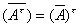§2  矩阵的运算

 运算及其规则 性质与说明 [相等]=当且仅当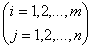相等矩阵必须具有相同行数与相同列数.     两矩阵相等，指各对应位置的元素分别相等. [加减]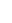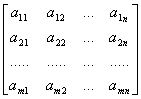±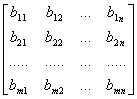=其中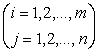同类型的矩阵才能相加减（各对应位置的元素相加减）. A+B=B+A      (交换律) (A+B)+C=A+(B+C)                  (结合律) [数乘]=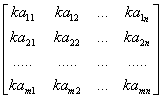数乘矩阵时，将数乘到矩阵的每个元素上. kA=Ak k(A+B)=kA+kB (k+l)A=kA+lA k(lA)=(kl)A (k,l为任意两个复数) [乘法] 若   A=(aij)为m´n矩阵   B=(bij)为n´s矩阵,则   AB=(aij) (bij)=(cij)=C 式中C为m´s矩阵，且 cij=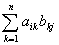运算及其规则 乘积的元素cij，等于左矩阵的第i行与右矩阵的第j列对应元素相乘之后相加. 左矩阵的列数必须等于右矩阵的行数. （AB）C=A（BC）    (结合律)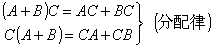k(AB)=(kA)B=A(kB)       (k是任意复数) [注]AB=BA一般情况下不成立，即无交换律.    性质与说明 [转置] 把m´n矩阵A=(aij)的列同行互换后所得到的n´m矩阵称为A的转置矩阵，记作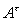,即=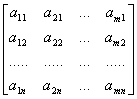(A+B)t=At+Bt (kA)t=kAt(k为任意复数) (AB)t=BtAt(反序定律) (A1A2...As)t=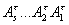(Ak)t=(At)k (k为整数) [共轭] 把矩阵A=(aij)的所有元素换成它们的共轭复数后所得到的矩阵称为A的共轭矩阵，记作，即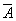=（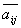）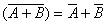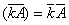(k为任意复数)